## David Sims

ID:2616
First Name:David
Last Name:Sims
Last Change:2020-06-06
Number of Files:6 (274th most prolific)

 ELLIPSES 1.0   (details) Prime ENG   2KB/3KB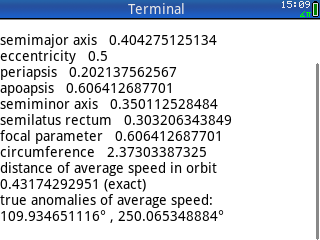User inputs the semimajor axis and eccentricity of an elliptical orbit. The code finds distances for periapsis, apoapsis, semiminor axis, semilatus rectum, focal parameter, circumference of orbit, and the separation between the primary focus and the orbiting object where the latter has its average speed in orbit relative to the former, and, finally, the true anomalies for this average speed. By David Sims. 2020/06/06

 MSSTARS 1.0   (details) Prime ENG   6KB/15KB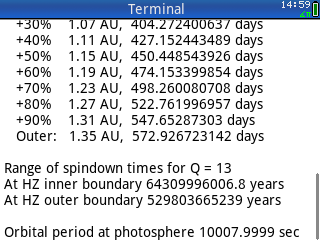A curvefit model for "average" population 1 main sequence stars. User enters the star's mass, all other parameters are calculated and displayed. By David Sims. 2020/06/06

 OE2PV 1.0   (details) Prime ENG   4KB/16KB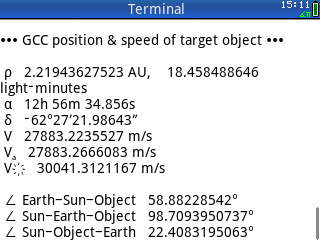Accepts as input the elements for a heliocentric elliptical or hyperbolic orbit, and a time of interest. The user inputs data by adjusting the values within the code. OE2PV outputs heliocentric state vectors for the object and for Earth, and it further outputs the geocentric distance, right ascension, and declination for the object. By David Sims. 2020/06/06

 ORBIT4 1.0   (details) Prime ENG   6KB/21KB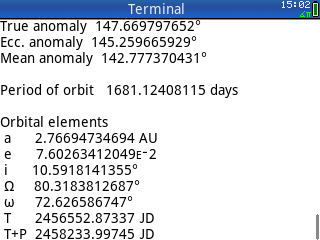A preliminary orbit determination code. By editing the code, the user enters position data for Earth and directional data for another object orbiting the sun at four distinct instants of observation. The program calculates the elements of the object's heliocentric orbit. By David Sims. 2020/06/06

 PLANCK 1.0   (details) Prime ENG   2KB/3KB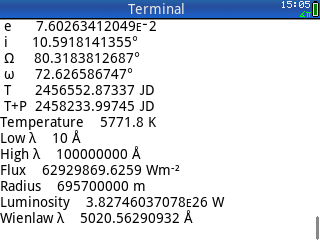Code that solves the Planck integral for radiant flux in watts per square meter, given a temperature in Kelvins, between a lower wavelength and an upper wavelength, with an option to calculate the luminous power of a sphere of input radius. By David Sims. 2020/06/06

 PLUNGE 1.0   (details) Prime ENG   5KB/8KB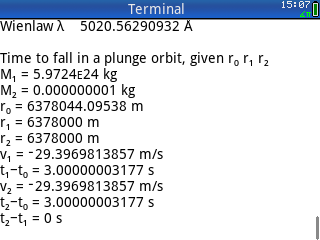Calculates the time-to-fall in a plunge orbit (eccentricity=. The zip file contains two programs. PLUNGE1 requires input values for the initial separation where two objects are at mutual rest, and for the separation between those same objects at two subsequent times prior to contact. The code calculates the amount of time to fall from the initial separation to each of the subsequent positions, and then it calculates the time difference; i.e. the amount of time to fall from the first subsequent position to the second subsequent position. PLUNGE2 requires input values for an observed separation and for an observed radial velocity (in approach) for that separation, and for a final separation. The initial distance is calculated using the Vis Viva equation. Then the amount of time required to fall from the initial separation to the observed separation is calculated. Next, the amount of time required to fall from the initial separation to the final separation is calculated. Finally the time difference required to fall from the observed position to the final position is found by subtraction. By David Sims. 2020/06/06

Part of the HP Calculator Archive,